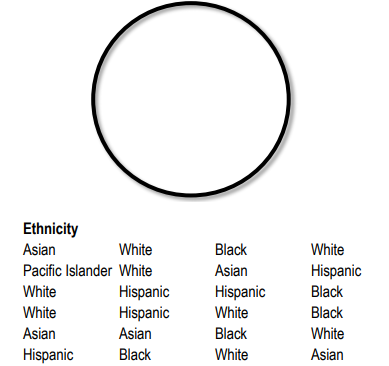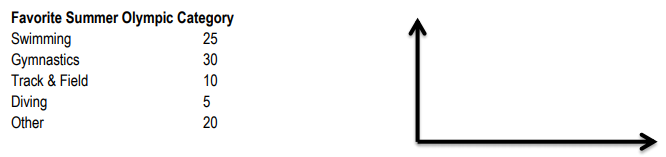Ch.1: Displaying Numeric DataWorksheetSee all chapters
 Ch.1: Displaying Numeric Data 1hr & 19mins 0% complete WorksheetDownload the video lesson worksheet Ch.2: Measures of Center and Spread 2hrs & 18mins 0% complete WorksheetDownload the video lesson worksheet Ch.3: Probability and Rules 1hr & 44mins 0% complete WorksheetDownload the video lesson worksheet Ch.4: The Discrete Random Variable 53mins 0% complete WorksheetDownload the video lesson worksheet Ch.5: The Binomial Random Variable 1hr & 38mins 0% complete WorksheetDownload the video lesson worksheet Ch.6: Types of Continuous Random Variable Distributions 1hr & 35mins 0% complete WorksheetDownload the video lesson worksheet Ch.7: The Standard Normal Distribution (Z-Scores) 1hr & 22mins 0% complete WorksheetDownload the video lesson worksheet Ch.8: Using The Z-Score 1hr & 24mins 0% complete WorksheetDownload the video lesson worksheet Ch.9: Sampling Distributions: Mean 1hr & 22mins 0% complete WorksheetDownload the video lesson worksheet Ch.10: Sampling Distributions: Proportion 1hr & 31mins 0% complete WorksheetDownload the video lesson worksheet Ch.11: Hypothesis Testing: Part 1 1hr & 42mins 0% complete WorksheetDownload the video lesson worksheet Ch.12: Hypothesis Testing: Part 2 1hr & 43mins 0% complete WorksheetDownload the video lesson worksheet

# Qualitative Data

See all sections
Sections
Qualitative Data
Quantitative Data

Concept #1: How To Display Qualitative Data

Concept #2: How To Display Qualitative Data Intro

Practice: Below are the ethnicities of the students in a particular Statistics class. Determine the relative frequency ofeach of the ethnicities.Practice: Construct a pie chart to represent the data below.Practice: Construct a bar graph for the following data set: